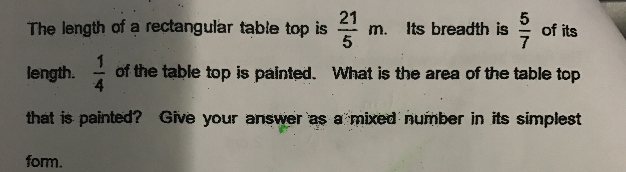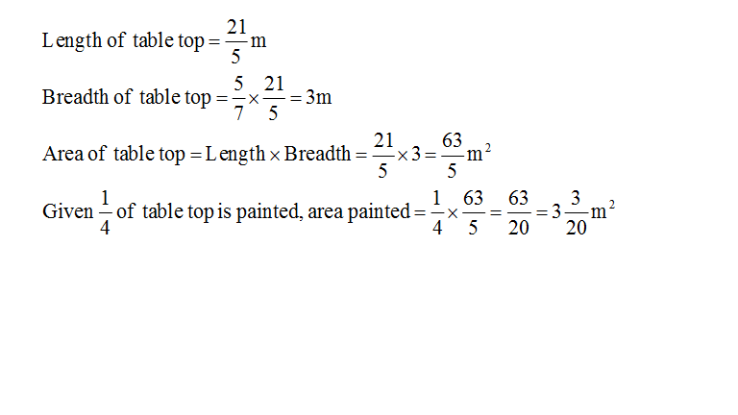# QuestionSource: Nanyang Primary0 Replies 1 Like ✔Accepted Answer

7u ——- 21/5 (length)
1u ——- (21/5)/7 = 21/35
5u ——- (21/35) x 5 = 21/7 = 3 (breadth)
(1/4) x (21/5) x 3 = 63/20 = 3 3/20

Ans : 3 3/20 sq m.

0 Replies 0 Likes

Area painted

= 1/4 x area of rectangle

= 1/4 x (L)    x (B)

= 1/4 x  (21/5) x (5/7 x 21/5)

= 3×21/ (4×5)

= 3  3/20 sq m

0 Replies 1 Like